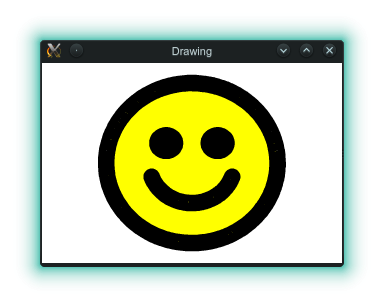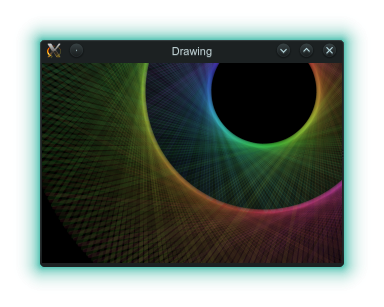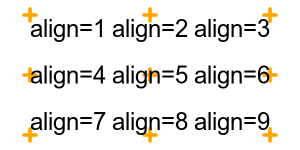# Drawing

4

1

4

2

### contributorsTests failing because of problems with Tk:## Warning:

Currently (Nov 2015) Julia graphics libs seem to be in a mess. I had a really bad time just getting basic dependencies installed and running. As far as I can tell this isn't my library's fault. Sorry!

Also, you need to use the development version of the Tk package to avoid deprecation errors (`Pkg.clone("git@github.com:JuliaLang/Tk.jl.git")` or similar).

# Introduction

A library for easy, extensible, drawing (diagrams, lines, shapes).

In other words: simple, imperative vector graphics; a wrapper around Cairo; an interface similar to Processing. For a declarative approach, see Compose.jl. For more control and complexity, use Cairo.jl directly.

In providing a simple API, the package has two important aims:

1. Changes to the graphics context are scoped. This is implemented via "do blocks".

2. Changes are nested and composable. So, for example, you can define a context with certain attributes (ink colour, pen width, etc), and then have an inner scope that changes a subset of those values.

# Examples

## Smiley Face

``````julia> Pkg.add("Drawing")

julia> using Drawing

julia> with(Axes(negative=true), Pen(0.2)) do
paint(Ink("yellow")) do
circle(1)           # face background, painted yellow
end
draw() do
circle(1)           # face outline, drawn black (default)
circle(0.5; from=200deg, to=340deg)    # smile
end
paint() do
move(0.3, 0.25)     # right eye position
circle(0.2)         # paint eye
move(-0.3, 0.25)    # left eye position
circle(0.2)         # paint eye
end
end
``````

which displays this window:## Transparent Colours

Pressing return and continuing...

``````julia> using ColorTypes
julia> r1, r2, r3, n, w = 1, 1, 0.5, 200, 0.5
julia> d1, d2, rotn = 120, -140, 40
julia> sat, val, alpha = 0.7, 0.5, 0.1
julia> width = 2*pi*r1 / n * w
julia> with(Axes(negative=true),
Translate(0.9, 0.9), Scale(3),
Paper("black"),
Pen(width; cap="round")) do
for x in shuffle!([i / n for i in 1:n])
hue = x * 360
draw(Ink(HSVA(hue, sat, val + (1-val)*rand(), alpha))) do
move(r1 * cos(-theta1), r1  * sin(-theta1))
line(r2 * cos(-theta2), r2  * sin(-theta2))
move(r1 * cos(-theta1), r1  * sin(-theta1))
line(r3 * cos(-theta3), r3  * sin(-theta3))
end
end
end
``````

displays## Other

• A chart showing evolution of a genetic algorithm over time.

# API

The API consists of:

1. Scopes introduce attributes, may include either nested scopes or actions, and may trigger some process (like stroking or filling a path) at the end. For example, `draw()` is a scope that introduces attributes, includes actions, and draws the path on closing.

2. Attributes modify the Cairo context for the duration of the scope. For example, `Ink()` sets the Cairo source.

3. Actions typically describe a Cairo path. For example, `move()` and `line()`.

These can be seen in the example below, which has three scopes, nested two deep, and includes the attributes `Ink()`, `Pen()`, etc.

``````with(PNG("red-blue-square.png", 100, 100), Ink("red"), Pen(0.1)) do
draw(Ink("blue")) do
move(0, 0)
line(1, 0)
line(1, 1)
end
draw() do  # the Ink is red here - the scope above has closed
line(0, 1)
line(0, 0)
end
end
``````The default coordinate system is from 0 to 1 (on the shorter axis, origin bottom left), with 10% of the space used as a border on each side. Unlike "raw" cairo, the "current point" is preserved across strokes.

## Scopes

• `with()` defines a scope, but takes no action on closing.

• `draw()` defines a scope and, on closing, draws the current path.

• `paint()` defines a scope and, on closing, fills the current path.

• `cairo()` should be followed by a do block with a single argument, which is the cairo context. This can then be used to make arbitrary calls to Cairo functions.

For example:

``````  julia> using Drawing

julia> using Cairo

julia> cairo() do ctx
line_to(ctx, 1, 1)
stroke(ctx)
end
``````

There are some constraints on how scopes are used: the `with()` scope cannot contain actions; the `draw()`, `paint()` and `cairo()` scopes cannot contain nested scopes.

## Attributes

There are two kinds of attribute: initial and dynamic.

The order of initial attributes is not important, but they can only appear in the outermost scope. The order of dynamic attributes may be important (eg the relative order of `Scale()` and `Translate()`) and follows the order given in the scope, from left to right.

### Initial Attributes

• `PNG(path, width_px, height_px)` initializes a Cairo context and, at the end of the scope, writes a PNG format image, of the given size, to the given path. Here width and height are measured in pixels.

• `PDF(path, width_mm, height_mm)` initializes a Cairo context and, at the end of the scope, writes a PDF format image, of the given size, to the given path. Here width and height are measured in mm.

• `PDF(path; size="a4", orientation="landscape")` initializes a Cairo context and, at the end of the scope, writes a PDF format image, of the given size, to the given path.

• `TK(width_px, height_px; name="Drawing", destroy=true)` initializes a Cairo context associated with a Tk window, which is displayed at the end of the scope. If `destroy=true` then the user is asked to press return, after which the window is destroyed; if it is false then there is no prompt and the window remains on the screen (until the window is closed by the user or julia exits).

• `Paper(background)` sets the background colour.

• `Axes(; scale=1, border=0.1, centred=true, negative=false)` Sets the Cairo user coordinates. Excluding the border (measured as a fraction of the smallest side), the coordinate system is either bottom left, or centred, and is scaled uniformly (ie assuming square pixels), so that the smallest axis has the given scale (length). If `negative=true` then the axis is from `-scale` to `scale`.

The `scale` can also be a tuple, `(nx, ny)`. In that case, the scale is chosen so that both those scales fit within the plotting area (but pixels remain square).

Below are plotted unit axes (x is horizontal) for 100x140 pixel images, with a border of 0.1 (ie 10 pixels), where the landscape axes extend to negative values. Note that drawing is not clipped to the unit axes area - it can extend over the whole page.

Click on image to see associated script.

### Dynamic Attributes

• `Ink(colour)` sets the Cairo source (ie the colour used to draw or paint). The colour can be a `Color` (see the Colors.jl package) or string ("red", "blue", etc).

• `Pen(width; cap=..., join=...)` sets the Cairo stroke properties (cap and join can be strings or constants from the Cairo.jl package). Omitted values are unchanged.

Note: pen width is in user units, but does not change if `Scale()` is used afterwards.

• `Pen(; cap=..., join=...)` as above, but allows width to be omitted (in which case the previous value is used).

Below, example lines with cap/join (from left to right) of round/round, butt/mitre, square/bevel.

Click on image to see associated script.

• `Scale(factor)` scales the Cairo user coordinates. This is a relative correction - values smaller than one mean that the same shape (ie the same coordinates) displays a smaller result.

Note: To scale the initial axes to have a range of 0-100, say, use `Scale(1/100)` (ie use the reciprocal of the range required).

• `Translate(x, y)` translates the Cairo user coordinates. This is a relative correction - the same shape (ie the same coordinates) appear shifted by the given x and y offsets.

Click on image to see associated script.

## Actions

Currently, all actions affect the Cairo path.

• `move(x, y)` moves the current point to given point in user coordinates.

• `line(x, y)` creates a straught line path between the current point and the given point, in user coordinates.

• `circle(radius; from=0, to=360)` create a circle centred at the current point, with a given radius. Setting `from` and `to` to smaller angles creates an arc (incomplete circle, with angles measured anti-clockwise from the x axis, in degrees).

• `rectangle(width, height; align=1, rotate=0)` create a rectangle, rotated and placed relative to the current point using the "telephone pad" align (1 is top left, 9 is bottom right).

• `square(side; align=1, rotate=0)` create a square, rotated and placed relative to the current point using the "telephone pad" align (1 is top left, 9 is bottom right).

• `text(s; align=1, rotate=0)` create the given text, rotated and placed relative to the current point using the "telephone pad" align (1 is top left, 9 is bottom right).

To clarify the `align` parameter, here are some text paths, align to the nearest orange cross.Click on image to see associated script.

# Changes

• Post 0.1: Changed `Axes()` so that what was `centred` is now `negative`, while what is now `centred` is really centred.

08/30/2015

11 months ago

197 commits